dsb (denoised and scaled by background) is an R package developed in John Tsang’s Lab for removing noise and normalizing protein data from single cell methods measuring protein with DNA-barcoded antibodies such as CITE-seq or Mission Bio Tapestri data. See the dsb Biorxiv preprint for details and please consider citing the paper if you use this package or find our experiments / modeling describing the sources of noise in CITE-seq data useful.

## Background and motivation

CITE-seq protein data suffers from substantial background noise (for example, see supplementary fig 5a in Stoeckius et. al. 2017 Nat. Methods). We performed experiments and analysis to dissect this noise; the dsb method is based on 3 key findings outlined in our preprint

1. Based on spike in experiments and modeling we found a major source of protein background noise comes from ambient, unbound antibody encapsulated in droplets.

2. “Empty” droplets (containing ambient mRNA and antibody but no cell), outnumber cell-containing droplets by 10-100 fold and capture the ambient component of protein background noise.

3. Cell-to-cell technical variations (i.e. stochastic differences in capture, RT efficiency, sequencing depth, and non-specific antibody binding) can be estimated and removed through a model of each cell’s “technical component” using isotype control counts and per cell mixture modeling.

## Installation and quick overview

Install dsb directly from CRAN using install.packages('dsb').

install.packages('dsb')

Use the DSBNormalizeProtein() function to normalize included package data of a raw protein count matrix cells_citeseq_mtx using background/empty droplet matrix empty_drop_citeseq_mtx. Model and remove the ‘technical component’ of each cell’s protein library by setting denoise.counts = TRUE including isotype controls in calculating the technical component with use.isotype.control = TRUE.

library(dsb)
# cell-containing droplet raw protein count matrix
cell_protein_matrix = cells_citeseq_mtx,
# empty/background droplet raw protein counts
empty_drop_matrix = empty_drop_citeseq_mtx,

# recommended step: model + remove the technical component of each cell's protein library
denoise.counts = TRUE,
use.isotype.control = TRUE,
isotype.control.name.vec = rownames(cells_citeseq_mtx)[67:70]
)

## Tutorial with public 10X Genomics data

Below we demonstrate dsb using data 10X Genomics data aligned with Cell Ranger. We define cells and background drops, run QC, normalize protein with dsb, cluster cells based on dsb normalized protein levels, visualize and interpret clustering results based on dsb values. Finally we use dsb values directly with Seurat’s Weighted Nearest Neighbor multimodal RNA + protein clustering algorithm. Wrappers are also provided to integrate dsb into AnnData (python) and Bioconductor (SingleCellExperiment) data structures (see table of contents).

Download both the filtered and raw count matrices from Cell Ranger from this public 10X Genomics CITE-seq data.

To follow code exactly below without changing paths, download and uncompress the files ‘Feature / cell matrix (filtered)’ and ‘Feature / cell matrix (raw)’ which will be automatically renamed filtered_feature_bc_matrix and raw_feature_bc_matrix and add them to a directory data/ in your working directory.

The filtered output is a subset of the raw output that are the cells estimated by Cell Ranger’s improved cell calling algorithm based on the EmptyDrops algorithm. One should always set the Cell Ranger --expect-cells argument roughly equal to the estimated cell recovery per lane based on number of cells loaded in the experiment. The raw output is all possible cell barcodes ranging from 500k-6M barcodes depending on the assay version. We will filter these barcodes to extract the major background signal. You can also estimate cells directly from count aligners such as CITE-seq-count (set the argument –cells to a value >2e5 to align background) or Kallisto.

Your working directory should now be structured:
data/
|_filtered_feature_bc_matrix
|_raw_feature_bc_matrix

## Step 2 Load RNA and protein alignment (Cell Ranger) data and define metadata

Here we use the convenience function from Seurat Read10X which will automatically detect multiple assays and create two element list Gene Expression and Antibody Capture.

# load packages used in this vignette
suppressMessages(library(dsb))
suppressMessages(library(Seurat))
suppressMessages(library(tidyverse))

# define a vector of cell-containing barcodes and remove them from unfiltered data
stained_cells = colnames(cells$Gene Expression) background = setdiff(colnames(raw$Gene Expression), stained_cells)

# split the data into separate matrices per assay
prot = raw$Antibody Capture rna = raw$Gene Expression

# create metadata of droplet QC stats used in standard scRNAseq processing
rna_size = log10(Matrix::colSums(rna))
prot_size = log10(Matrix::colSums(prot))
ngene = Matrix::colSums(rna > 0)
mtgene = grep(pattern = "^MT-", rownames(rna), value = TRUE)
propmt = Matrix::colSums(rna[mtgene, ]) / Matrix::colSums(rna)
md = as.data.frame(cbind(propmt, rna_size, ngene, prot_size))
md$bc = rownames(md) md$droplet_class = ifelse(test = mdbc %in% stained_cells, yes = 'cell', no = 'background') # filter barcodes to only include those with data for both assays md = md %>% dplyr::filter(rna_size > 0 & prot_size > 0 ) ## Step 3 Quality control on cell-containing and background droplets The plot below shows the number of detected genes vs the protein library size for cells vs background drops. One can also define the cells vs background drops directly by thresholding on a plot like this if for example using a different count aligner that does not provide filtered cell output. ggplot(md, aes(x = log10(ngene), y = prot_size )) + theme_bw() + geom_bin2d(bins = 300) + scale_fill_viridis_c(option = "C") + facet_wrap(~droplet_class)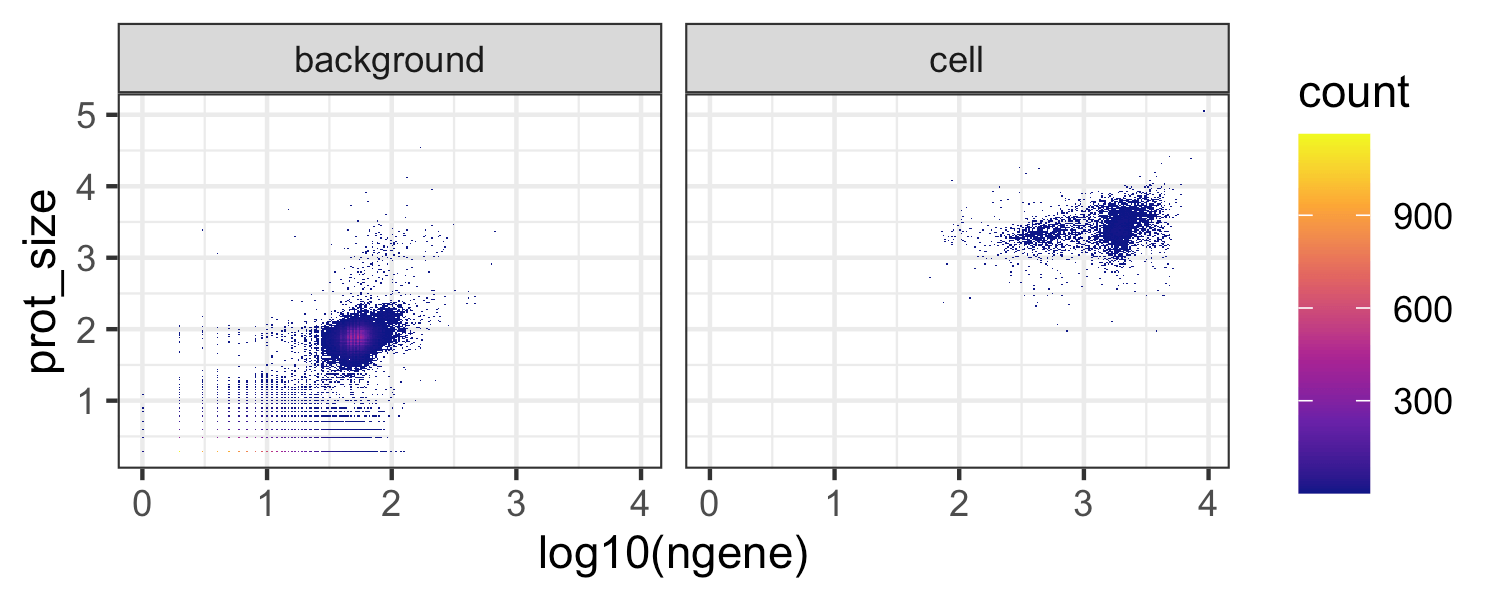We next further filter cells based on thresholds calculated from quality control metrics as in any standard scRNAseq analysis, e.g. see Luecken et. al. 2019 Mol Syst Biol.  cellmd = md %>% filter(droplet_class == 'cell') plot_aes = list(theme_bw(), geom_point(shape = 21 , stroke = 0, size = 0.7), scale_fill_viridis_c(option = "C")) p1 = ggplot(cellmd, aes(x = rna_size )) + geom_histogram(bins = 50) + theme_bw() + xlab("log10 RNA library size") p2 = ggplot(cellmd, aes(x = propmt)) + geom_histogram(bins = 50) + theme_bw() + xlab("mitochondrial read proportion") p3 = ggplot(cellmd, aes(x = log10(ngene), y = rna_size, fill = propmt )) + plot_aes p4 = ggplot(cellmd, aes(x = ngene, y = prot_size, fill = propmt )) + plot_aes p1+p2+p3+p4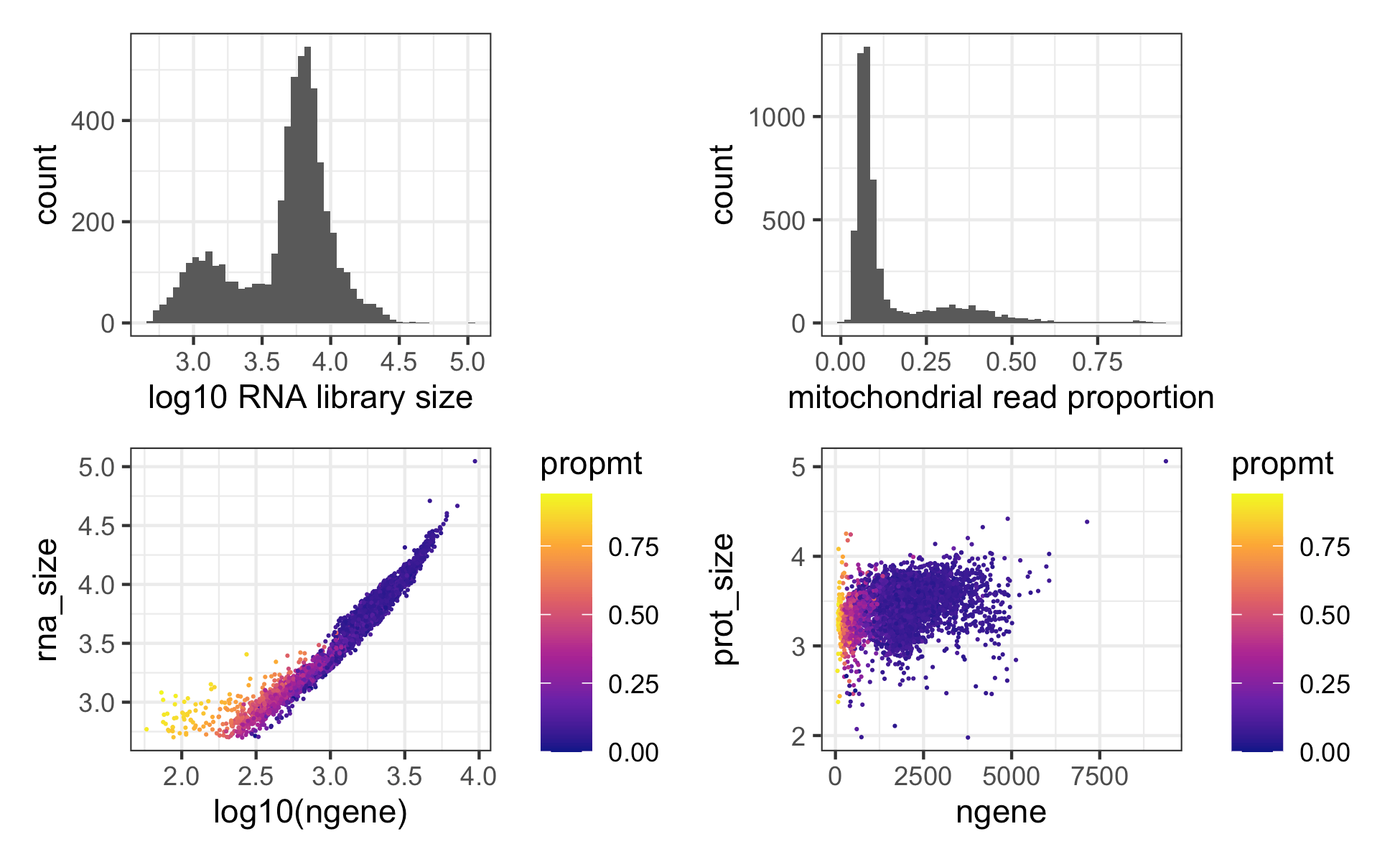Note cells with the smaller library size are mostly naive CD4 T cells which are small in size and naturally have less mRNA content. # calculate statistical thresholds for droplet filtering. rna_size_min = median(cellmdrna_size) - (3*mad(cellmd$rna_size)) rna_size_max = median(cellmd$rna_size) + (3*mad(cellmd$rna_size)) prot_size_min = median(cellmd$prot_size) - (3*mad(cellmd$prot_size)) prot_size_max = median(cellmd$prot_size) + (3*mad(cellmd$prot_size)) # filter rows based on droplet qualty control metrics positive_cells = cellmd[ cellmd$prot_size > prot_size_min &
cellmd$prot_size < prot_size_max & cellmd$propmt < 0.14 &
cellmd$rna_size > rna_size_min & cellmd$rna_size < rna_size_max, ]$bc cells_mtx_rawprot = as.matrix(prot[ , positive_cells]) Sanity check: are the number of QCd cells in line with the expected recovery from the experiment? length(positive_cells)  4096 Yes. After quality control above we have 4096 cells which is in line with the ~5000 cells loaded in this experiment. We also filter background droplets to remove potential spurious cells (drops with high mRNA content) and use the major peak in the background distribution between 1.4 and 2.5 log total protein counts. # define a vector of background droplet barcodes based on protein library size and mRNA content background_drops = md[md$prot_size > 1.5 & md$prot_size < 3 & md$ngene < 100, ]$bc negative_mtx_rawprot = as.matrix(prot[ , background_drops]) ## Optional step; remove proteins without staining While dsb will handle noisy proteins, some proteins in an experiment may not work for bioinformatic reasons or may target a very rare cell population that was absent in the experiment. This is especially true as panels increase in size. Proteins without counts on the stained cells should be removed prior to normalization. # calculate quantiles of the raw protein matrix d1 = data.frame(pmax = apply(cells_mtx_rawprot, 1, max)) %>% rownames_to_column('prot') %>% arrange(pmax) %>% head()  prot pmax CD34_TotalSeqB 4 CD80_TotalSeqB 60 CD274_TotalSeqB 75 IgG2b_control_TotalSeqB 90 IgG2a_control_TotalSeqB 95 IgG1_control_TotalSeqB 112 In this experiment, the stem cell marker CD34 has essentially no data (a maximum UMI of 4 across all cells in the experiment). We therefore remove it from cell and background matrices. In many cases, removing proteins is not necessary. # remove non staining CD34 protein prot_names = rownames(cells_mtx_rawprot) cells_mtx_rawprot = cells_mtx_rawprot[!prot_names == 'CD34_TotalSeqB', ] negative_mtx_rawprot = negative_mtx_rawprot[!prot_names == 'CD34_TotalSeqB', ] ## Step 4 Normalize protein data with the DSBNormalizeProtein Function For data with isotype control proteins, set denoise.counts = TRUE and use.isotype.control = TRUE and provide a vector containing names of isotype control proteins (the rownames of the protein matrix that are isotype controls). For data without isotype controls, see the vignette section Using dsb with data lacking isotype controls. #normalize protein data for the cell containing droplets with the dsb method. dsb_norm_prot = DSBNormalizeProtein( cell_protein_matrix = cells_mtx_rawprot, empty_drop_matrix = negative_mtx_rawprot, denoise.counts = TRUE, use.isotype.control = TRUE, isotype.control.name.vec = rownames(cells_mtx_rawprot)[29:31] ) # note: normalization takes ~ 20 seconds # system.time() # user system elapsed # 20.799 0.209 21.783  The function returns a matrix of normalized protein values which can be integrated with any single cell analysis software. We provide an example with Seurat, Bioconductor and Scanpy below. optional QC step sometimes denoising results in very small negative values for a protein corresponding to very low expression; it can be helpful to convert all dsb normalized values < -10 to 0 for interpretation / visualization purposes. (see FAQ) dsb_norm_prot = apply(dsb_norm_prot, 2, function(x){ ifelse(test = x < -10, yes = 0, no = x)})  ## Integrating dsb with Seurat # filter raw protein, RNA and metadata to only include cell-containing droplets cells_rna = rna[ ,positive_cells] md2 = md[positive_cells, ] # create Seurat object !note: min.cells is a gene filter, not a cell filter s = Seurat::CreateSeuratObject(counts = cells_rna, meta.data = md2, assay = "RNA", min.cells = 20) # add dsb normalized matrix "dsb_norm_prot" to the "CITE" assay data slot s[["CITE"]] = Seurat::CreateAssayObject(data = dsb_norm_prot) This object can be used in downstream analysis using Seurat (note to use dsb values do not use the default CLR normalization after adding dsb values). ## Clustering cells based on dsb normalized protein using Seurat Here we will cluster the cells and annotate them based on dsb normalized protein levels. This is similar to the workflows used in our paper Kotliarov et. al. 2020 Nat. Medicine. We first run spectral clustering using Seurat directly on the dsb normalized protein values without reducing dimensionality of the cells x protein matrix with PCA. # cluster and run umap (based directly on dsb normalized values without istype controls ) prots = rownames(s@assays$CITE@data)[1:28]

s = FindNeighbors(object = s, dims = NULL, assay = 'CITE',
features = prots, k.param = 30, verbose = FALSE)

# direct graph clustering
s = FindClusters(object = s, resolution = 1, algorithm = 3, graph.name = 'CITE_snn', verbose = FALSE)

# umap (optional)
s = RunUMAP(object = s, assay = "CITE", features = prots, seed.use = 1990,
min.dist = 0.2, n.neighbors = 30, verbose = FALSE)

## dsb derived cluster interpretation

A heatmap of average dsb normalized values in each cluster help in annotating clusters results. The values for each cell represent the number of standard deviations of each protein from the expected noise from reflected by the protein’s distribution in empty droplets, +/- the residual of the fitted model to the cell-intrinsic technical component.

# make results dataframe
d = cbind(s@meta.data, as.data.frame(t(s@assays$CITE@data)), s@reductions$umap@cell.embeddings)

# calculate the median protein expression separately for each cluster
dplyr::group_by(CITE_snn_res.1) %>%
dplyr::summarize_at(.vars = prots, .funs = median) %>%
tibble::remove_rownames() %>%
tibble::column_to_rownames("CITE_snn_res.1")
# plot a heatmap of the average dsb normalized values for each cluster
color = viridis::viridis(25, option = "B"),
fontsize_row = 8, border_color = NA)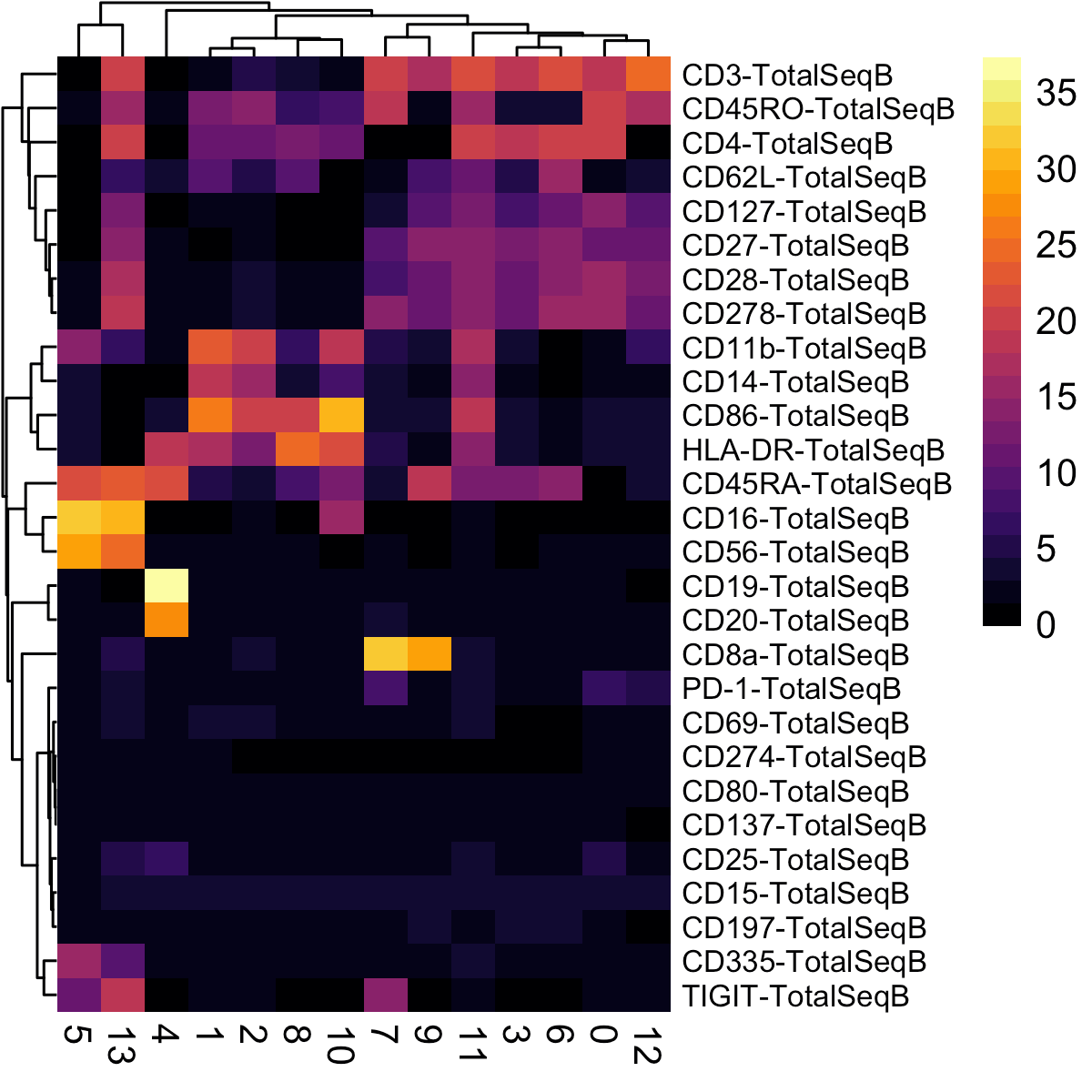Annotate cell types based on median dsb normalized protein levels per cluster


clusters = c(0:13)
celltype = c("CD4_Tcell_Memory", # 0
"CD14_Monocytes", #1
"CD14_Monocytes_Activated", #2
"CD4_Naive_Tcell", #3
"B_Cells", #4
"NK_Cells", #5
"CD4_Naive_Tcell_CD62L+", #6
"CD8_Memory_Tcell", #7
"DC", #8
"CD8_Naive_Tcell", #9
"CD4_Effector", #10
"CD16_Monocyte", #11
"DOUBLETS", #12
"DoubleNegative_Tcell" #13
)
s@meta.data$celltype = plyr::mapvalues(x = s@meta.data$CITE_snn_res.1, from = clusters, to = celltype)

# plot
Seurat::DimPlot(s, reduction = 'umap', group.by = 'celltype',
label = TRUE, repel = TRUE, label.size = 2.5, pt.size = 0.1) +
theme_bw() + NoLegend() + ggtitle('dsb normalized protein')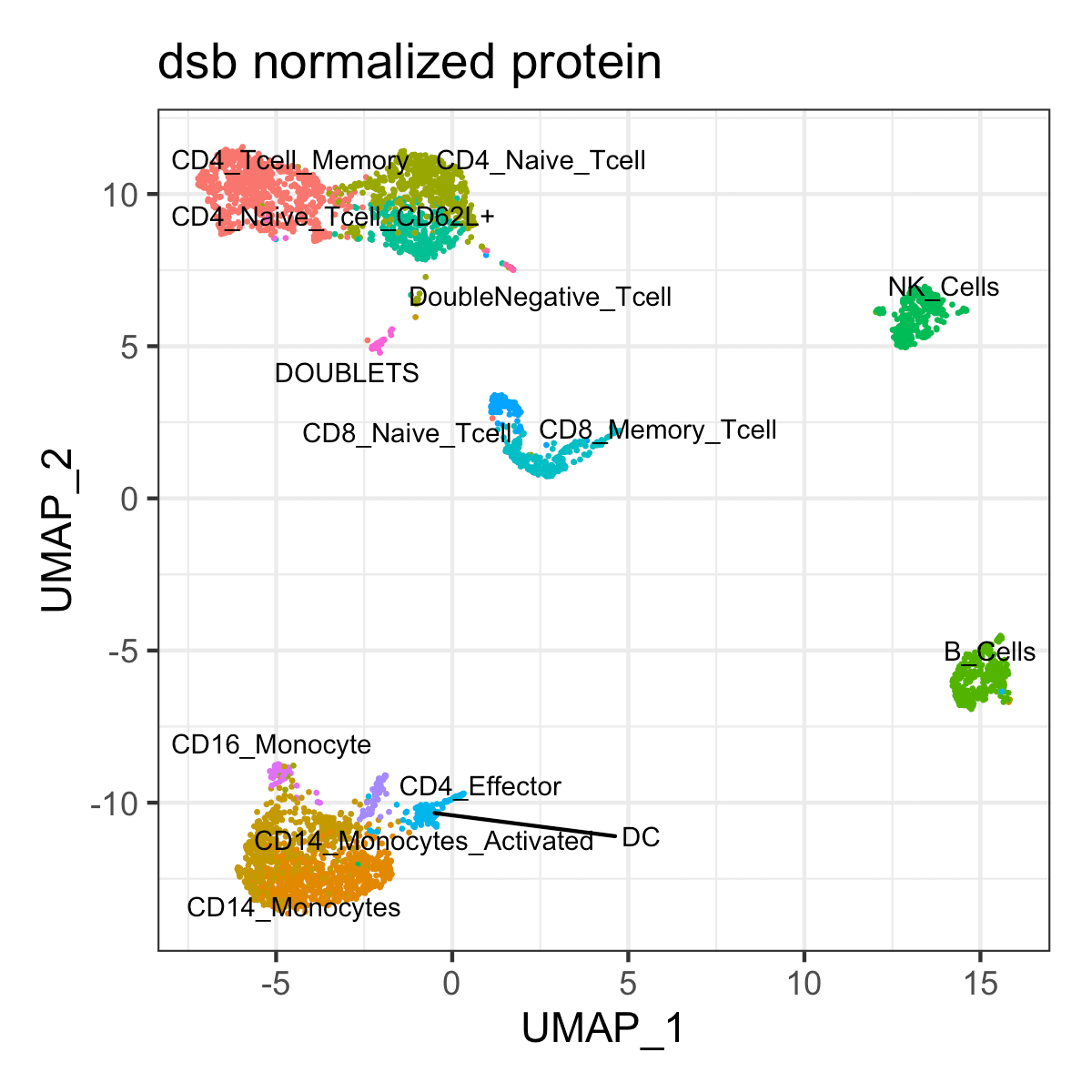## Weighted Nearest Neighbor multimodal clustering using dsb normalized values with Seurat

Below we demonstrate using Seurat’s weighted nearest neighbors multimodal clustering method with dsb normalized values as input for the protein assay. The performance of this algorithm is better on larger datasets but we demonstrate here on this small dataset as an example.

Of note, the default recommendation of the WNN method is to first compress both the ADT and mRNA data into principal components. For a dataset with a smaller number of proteins, we have found that just using the dsb normalized cells x protein directly rather than compressing the ADT data into principal components can improve the resulting clusters and interpretation of the joint embedding. Datasets generated with recently available pre-titrated panels consisting of more than 100 or 200 proteins may benefit more from dimensionality reduction with PCA.

Below, examples are provided for using both the dsb normalized protein values directly as well as the dsb normalized values compressed into principal components (the default WNN method). Again, this algorithm is more powerful on datasets with a greater number of cells.

# use pearson residuals as normalized values for pca
DefaultAssay(s) = "RNA"
s = NormalizeData(s, verbose = FALSE) %>%
FindVariableFeatures(selection.method = 'vst', verbose = FALSE) %>%
ScaleData(verbose = FALSE) %>%
RunPCA(verbose = FALSE)

# set up dsb values to use in WNN analysis
DefaultAssay(s) = "CITE"
# hack seurat to use normalized protein values as a dimensionality reduction object.
VariableFeatures(s) = prots

# run true pca to initialize dr pca slot for WNN
s = ScaleData(s, assay = 'CITE', verbose = FALSE)
s = RunPCA(s, reduction.name = 'pdsb', features = VariableFeatures(s), verbose = FALSE)

# make matrix of norm values to add as dr embeddings
pseudo = t(s@assays$CITE@data)[,1:29] pseudo_colnames = paste('pseudo', 1:29, sep = "_") colnames(pseudo) = pseudo_colnames # add to object s@reductions$pdsb@cell.embeddings = pseudo

# run WNN
s = FindMultiModalNeighbors(
object = s,
reduction.list = list("pca", "pdsb"),
weighted.nn.name = "dsb_wnn",
knn.graph.name = "dsb_knn",
modality.weight.name = "dsb_weight",
snn.graph.name = "dsb_snn",
dims.list = list(1:30, 1:29),
verbose = FALSE
)

s = FindClusters(s, graph.name = "dsb_knn", algorithm = 3, resolution = 1.5,
random.seed = 1990,  verbose = FALSE)
s = RunUMAP(s, nn.name = "dsb_wnn", reduction.name = "dsb_wnn_umap",
reduction.key = "dsb_wnnUMAP_", seed.use = 1990, verbose = FALSE)

# plot
p1 = Seurat::DimPlot(s, reduction = 'dsb_wnn_umap', group.by = 'dsb_knn_res.1.5',
label = TRUE, repel = TRUE, label.size = 2.5, pt.size = 0.1) +
theme_bw() +
xlab('dsb protein RNA multimodal UMAP 1') +
ylab('dsb protein RNA multimodal UMAP 2') +
ggtitle('WNN using dsb normalized protein values')

p1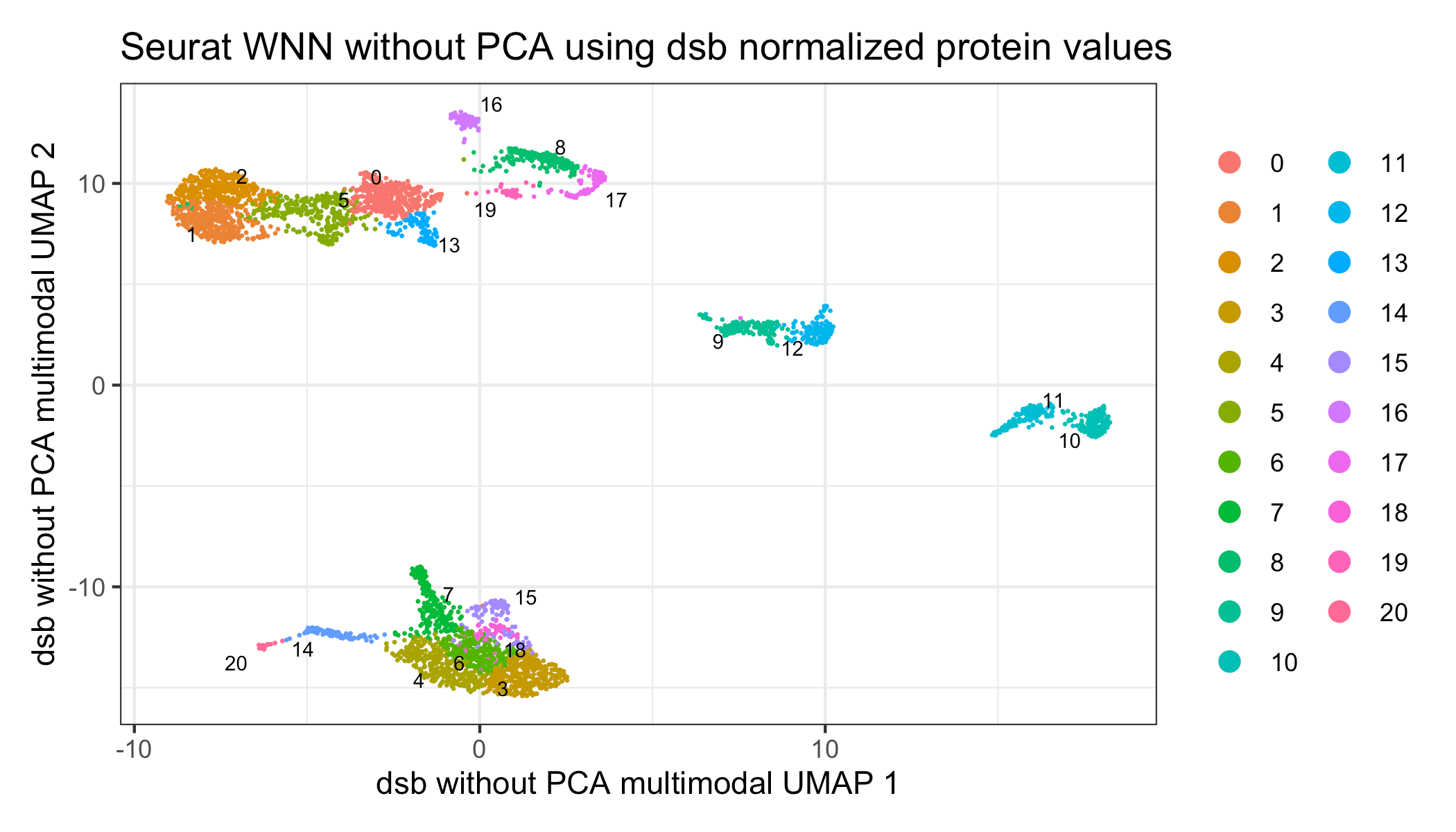Now we can add these higher resolution subcluster annotations to the dsb derived protein only clusters for interpretation and visualize the results with a multimodal heatmap.

# create multimodal heatmap
vf = VariableFeatures(s,assay = "RNA")

Idents(s) = "dsb_knn_res.1.5"
DefaultAssay(s)  = "RNA"
rnade = FindAllMarkers(s, features = vf, only.pos = TRUE)
gene_plot = rnade %>% filter(avg_log2FC > 1 ) %>%  group_by(cluster) %>% top_n(3) %$% gene %>% unique s@meta.data$celltype_subcluster = paste(s@meta.data$celltype, s@meta.data$dsb_knn_res.1.5)

d = cbind(s@meta.data,
# protein
as.data.frame(t(s@assays$CITE@data)), # mRNA as.data.frame(t(as.matrix(s@assays$RNA@data[gene_plot, ]))),
s@reductions$umap@cell.embeddings) # combined data adt_plot = d %>% dplyr::group_by(dsb_knn_res.1.5) %>% dplyr::summarize_at(.vars = c(prots, gene_plot), .funs = median) %>% tibble::remove_rownames() %>% tibble::column_to_rownames("dsb_knn_res.1.5") # make a combined plot suppressMessages(library(ComplexHeatmap)) # protein heatmap prot_col = circlize::colorRamp2(breaks = seq(-10,30, by = 2), colors = viridis::viridis(n = 18, option = "B", end = 0.95)) p1 = Heatmap(t(adt_plot)[prots, ], name = "protein",col = prot_col, use_raster = T, row_names_gp = gpar(color = "black", fontsize = 5)) # mRNA heatmap mrna = t(adt_plot)[gene_plot, ] rna_col = circlize::colorRamp2(breaks = c(-2,-1,0,1,2), colors = colorspace::diverge_hsv(n = 5)) p2 = Heatmap(t(scale(t(mrna))), name = "mRNA", col = rna_col, use_raster = T, clustering_method_columns = 'average', column_names_gp = gpar(color = "black", fontsize = 7), row_names_gp = gpar(color = "black", fontsize = 5)) ht_list = p1 %v% p2 draw(ht_list)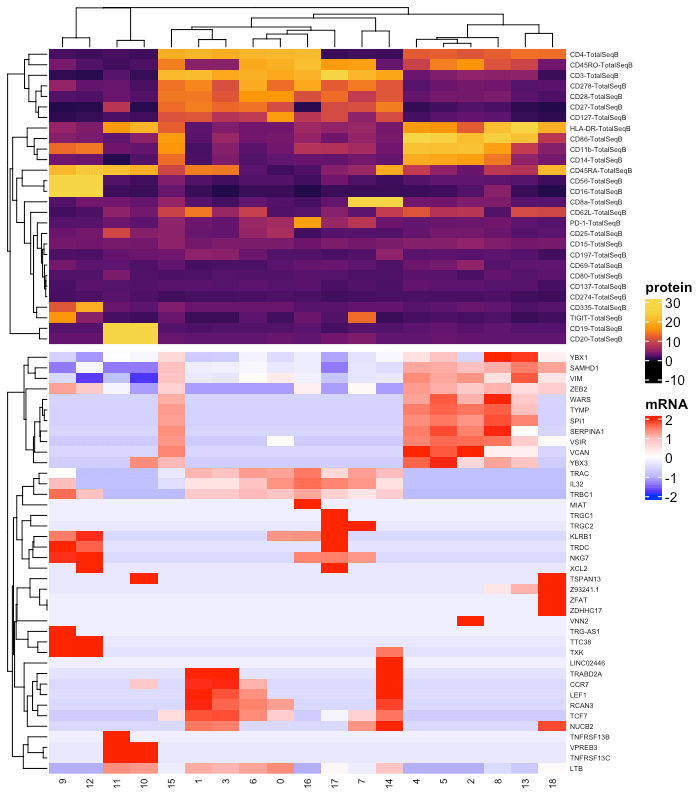One can also use the default implementation of Seurat’s WNN analysis with principal components based dsb normalized values as input. # use pearson residuals as normalized values for pca DefaultAssay(s) = "RNA" s = NormalizeData(s, verbose = FALSE) %>% FindVariableFeatures(selection.method = 'vst', verbose = FALSE) %>% ScaleData(verbose = FALSE) %>% RunPCA(verbose = FALSE) # set up dsb values to use in WNN analysis (do not normalize with CLR, use dsb normalized values) DefaultAssay(s) = "CITE" VariableFeatures(s) = prots s = s %>% ScaleData() %>% RunPCA(reduction.name = 'apca') # run WNN s = FindMultiModalNeighbors( s, reduction.list = list("pca", "apca"), dims.list = list(1:30, 1:18), modality.weight.name = "RNA.weight" ) # cluster s <- RunUMAP(s, nn.name = "weighted.nn", reduction.name = "wnn.umap", reduction.key = "wnnUMAP_") s <- FindClusters(s, graph.name = "wsnn", algorithm = 3, resolution = 1.5, verbose = FALSE, random.seed = 1990) p1 = Seurat::DimPlot(s, reduction = 'wnn.umap', group.by = 'wsnn_res.1.5', label = TRUE, repel = TRUE, label.size = 2.5, pt.size = 0.1) + theme_bw() + xlab('Default WNN with dsb values UMAP 1') + ylab('Default WNN with dsb values UMAP 2') p1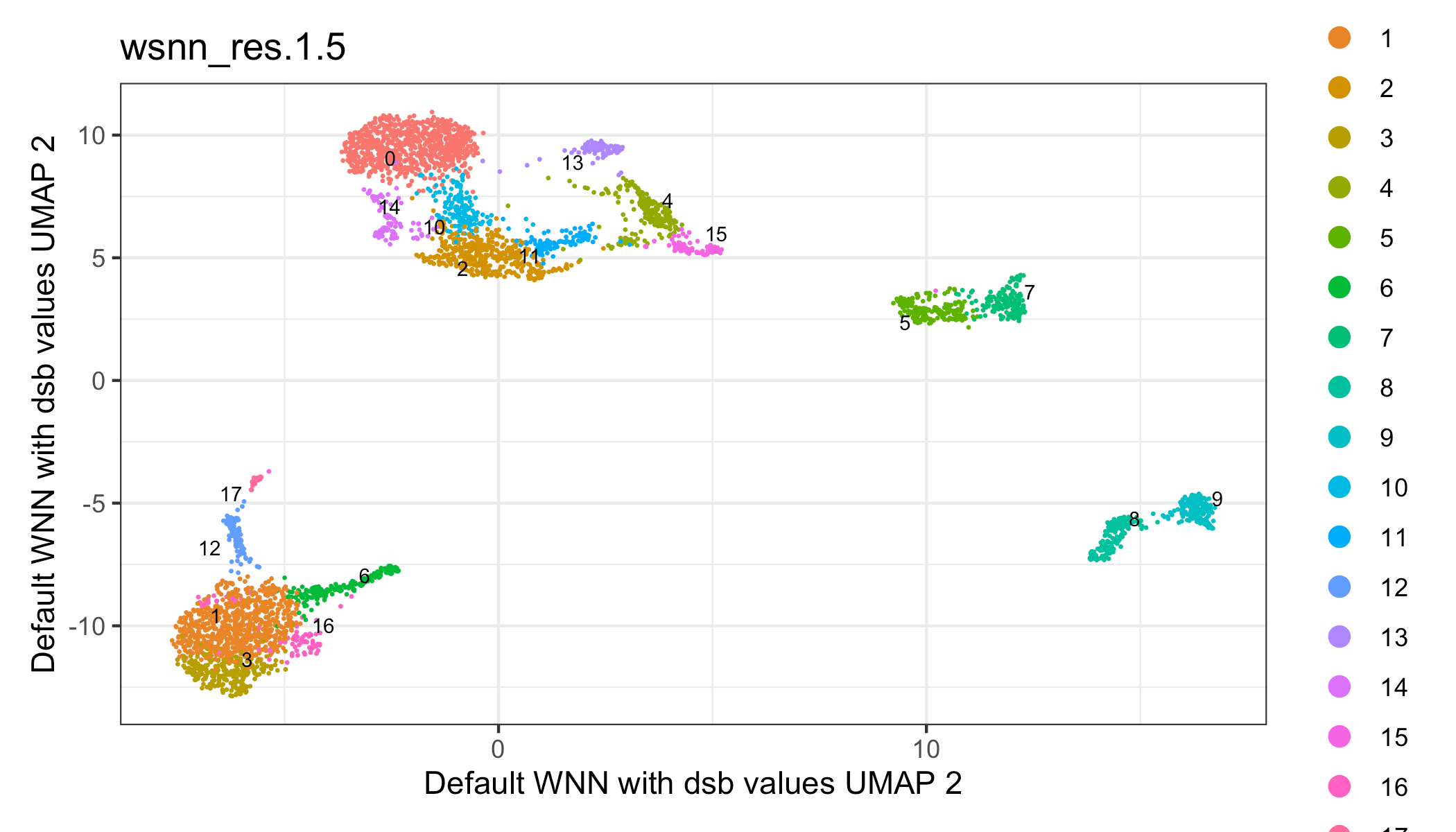## Integrating dsb with Bioconductor Rather than Seurat you may wish to use the SingleCellExperiment class to use Bioconductor packages - this can be accomplished with this alternative to step III above using the following code. To use Bioconductor’s semantics, we store raw protein values in an ‘alternative Experiment’ in a SingleCellExperiment object containing RNA counts. suppressMessages(library(SingleCellExperiment)) sce = SingleCellExperiment(assays = list(counts = cells_rna), colData = md2) # define the dsb normalized values as logcounts to use a common SingleCellExperiment / Bioconductor convention adt = SummarizedExperiment(assays = list('counts' = as.matrix(cells_mtx_rawprot), 'logcounts' = as.matrix(dsb_norm_prot))) altExp(sce, "CITE") = adt ## Integrating dsb with python/Scanpy You can also use dsb normalized values with the AnnData class in Python by following steps 1 and 2 above to load data, define drops and normalize with dsb in R. The simplest option is then to use reticulate to create the AnnData object from dsb denoised and normalized protein values as well as raw RNA data. That object can then be imported into a python session. Anndata are not structured as separate assays; we therefore need to merge the RNA and protein data. See the current Scanpy CITE-seq workflow and more on interoperability between Scanpy Bioconductor and Seurat NEW: dsb users keen on using python are also encouraged to checkout the dsb wrapper available in the muon python multimodal framework about muon dsb wrapper for python in muon library(reticulate); sc = import("scanpy") # merge dsb-normalized protein and raw RNA data combined_dat = rbind(count_rna, dsb_norm_prot) s[["combined_data"]] = CreateAssayObject(data = combined_dat) # create Anndata Object adata_seurat = sc$AnnData(
X   = t(GetAssayData(s,assay = "combined_data")),
obs = seurat@meta.data,
var = GetAssay(seurat)[[]]
)

## Using dsb with data lacking isotype controls

If isotype controls are not included, you can run dsb correcting ambient background without cell denoising. We only recommend setting denoise.counts = FALSE if isotype controls were not included in the experiment which results in not defining the technical component of each cell’s protein library. The values of the normalized matrix returned are the number of standard deviations above the expected ambient noise captured by empty droplets.

# suggested workflow if isotype controls are not included
dsb_rescaled = DSBNormalizeProtein(cell_protein_matrix = cells_citeseq_mtx,
empty_drop_matrix = empty_drop_citeseq_mtx,
# do not denoise each cell's technical component
denoise.counts = FALSE)

We strongly recommend using isotype controls, however if these are not available, the background mean for each cell inferred via a per-cell gaussian mixture model (µ1) can theoretically be used alone to define the cell’s technical component, however this assumes the background mean has no expected biological variation. In our data the background mean had weak but significant correlation with the foreground mean (µ2) across single cells (see the paper). Isotype controls anchor the component of the background mean associated with noise.


dsb_rescaled = dsb::DSBNormalizeProtein(cell_protein_matrix = cells_citeseq_mtx,
empty_drop_matrix = empty_drop_citeseq_mtx,
# denoise with background mean only
denoise.counts = TRUE,
use.isotype.control = FALSE)

## Integrating dsb with sample multiplexing experiments

In multiplexing experiments with cell superloading, demultiplexing functions define a “negative” cell population which can then be used to define background droplets for dsb. Multiplexing / Demultiplexing methods and functions compatible with dsb:
HTODemux (Seurat)
deMULTIplex (Multiseq)
demuxlet

In our data, dsb normalized values were nearly identically distributed when dsb was run with background defined by demultiplexing functions or protein library size (see the paper).

We load the raw output from cell ranger. Prior to demultiplexing we use the min.genes argument in the Seurat::Read10X function to partially threshold out some background drops yet still retain sufficient (often > 80,000 droplets per 10X lane depending on experiment) from which to estimate the background. This balances memory strain when demultiplexing tens of thousands of cells with requirements of the Seurat::HTODemux function to have sufficient empty drops to estimate the background population of each Hash antibody. Importantly, the HTODemux function may not converge if the cell ranger filtered output was loaded since there will not be sufficient negative drops to estimate the background for each hashing antibody. Increasing the number of drops used in demultiplexing will result in more droplets defined by the function as “negative” which can increase the confidence in the estimate of background used by dsb.

# raw = Read10X see above -- path to cell ranger outs/raw_feature_bc_matrix ;

# partial thresholding to slightly subset negative drops include all with 5 unique mRNAs
seurat_object = CreateSeuratObject(raw, min.genes = 5)

# demultiplex (positive.quantile can be tuned to dataset depending on size)
seurat_object = HTODemux(seurat_object, assay = "HTO", positive.quantile = 0.99)
Idents(seurat_object) = "HTO_classification.global"

# subset empty drop/background and cells
neg_object = subset(seurat_object, idents = "Negative")
singlet_object = subset(seurat_object, idents = "Singlet")

# non sparse CITEseq data store more efficiently in a regular matrix
neg_adt_matrix = GetAssayData(neg_object, assay = "CITE", slot = 'counts') %>% as.matrix()
positive_adt_matrix = GetAssayData(singlet_object, assay = "CITE", slot = 'counts') %>% as.matrix()

# normalize the data with dsb
dsb_norm_prot = DSBNormalizeProtein(
cell_protein_matrix = cells_mtx_rawprot,
empty_drop_matrix = negative_mtx_rawprot,
denoise.counts = TRUE,
use.isotype.control = TRUE,
isotype.control.name.vec = rownames(cells_mtx_rawprot)[30:32])

# now add the normalized dat back to the object (the singlets defined above as "object")
singlet_object[["CITE"]] = CreateAssayObject(data = dsb_norm_prot)

# proceed with same tutorial workflow shown above. 

Can other count aligners like Kallisto and CITE-seq-count be used? Yes! dsb was developed prior to 10X Genomics supporting CITE-seq or hashing data and we routinely use other alignment pipelines. It is important to specify a large number of cells for e.g. CITE-seq-count in order to make sure you obtain background reads and not just cells. If you loaded 10,000 cells, you would NOT set the –cells argument in CITE-seq-count to be 10000, you would set it to e.g. 300000 in order to align to background drops as well as the large library size cell-containing droplets. dsb can then be used exactly as in the vignette, one simply loads the ADT count data and defines foreground and background drops using thresholds based on mRNA and protein as shown in the vignette. For using Cell Ranger, the analogous argument expect-cells should actually be set to the number of expected recovered cells-Cell Ranger uses the EmptyDrops algorithm to call cells vs empty drops based in part on this parameter and returns the full output in the raw and just the estimated cells in the filtered outputs respectively (as shown in the documentation example above).

For comparison of CITE-seq alignment pipelines in a workflow that used dsb see: https://github.com/Terkild/CITE-seq_optimization

I get the error “Error in quantile.default(x, seq(from = 0, to = 1, length = n)): missing values and NaN’s not allowed if ‘na.rm’ is FALSE” What should I do? - This error occurs during denoising, (denoise = TRUE) when you have antibodies with 0 counts or close to 0 across all cells. To get rid of this error, check the distributions of the antibodies with e.g. apply(cells_protein_matrix, 1, quantile) to find the protein(s) with basically no counts, then remove these from the empty drops and the cells. Please refer to these links:
https://github.com/niaid/dsb/issues/6
https://github.com/niaid/dsb/issues/26

I get a "problem too large or memory exhausted error when I try to convert to a regular R matrix - (see issue 10 on the dsb github) CITE-seq protein counts don’t need a sparse representation-very likely this error is because there are too many negative droplets defined (i.e. over 1 million). You should be able to normalize datasets with 100,000+ cells and similar numbers of negative droplets (or less) on a normal 16GB laptop. By further narrowing in on the major background distribution, one should be able to convert the cells and background to a normal R matrix which should run successfully.
https://github.com/niaid/dsb/issues/10

the range of dsb normalized values is large is this normal? In all cases encountered thus far, the large range of values for a protein (e.g. ranging from -50 to 50) are caused by just a few outlier cells, most often a few cells with low negative values for the protein. We have now provided a quantile clipping option in dsb to address these outlier cells. Users can also investigate these cells to see if they have very high values for isotype control proteins and they can possibly be removed from the dataset. https://github.com/niaid/dsb/issues/22
https://github.com/niaid/dsb/issues/9

### check for outliers in dsb normalized values

# find outliers
pheatmap::pheatmap(apply(dsb_norm_prot, 1, function(x){
quantile(x,c(0.9999, 0.99, 0.98, 0.95, 0.0001, 0.01, 0.1))
}))

### implement dsb with Quantile clipping

By default setting quanitle.clipping = TRUE sets cells values for a given protein above the 99.95th percentile or below the 0.1 percentile of that protein’s expression to be thees quantile values. That value is optimized to remove a few high and low magnitude outliers but can be set to adapt to the number of cells in the dataset.

dsb_norm_prot = DSBNormalizeProtein(
cell_protein_matrix = cells_citeseq_mtx,
empty_drop_matrix = empty_drop_citeseq_mtx,
denoise.counts = TRUE,
use.isotype.control = TRUE,
isotype.control.name.vec = rownames(cells_citeseq_mtx)[67:70],
# implement Quantile clipping
quantile.clipping = TRUE,
# high and low otlier quantile across proteins to clip
quantile.clip = c(0.001, 0.9995)
)

### A simpler alternative to quantile clipping not requiring re-running dsb

Another simple option if there are a few low magnitude outliers: cells with a given protein value < -10 can be interpreted as not expressed. One can simply convert these values to 0 with the code below.

dsb_norm_prot = apply(dsb_norm_prot, 2, function(x){
ifelse(test = x < -10, yes = 0, no = x)
})

What are the minimum number of proteins required to use dsb dsb is compatible with datasets with any number of proteins with step I alone. We have validated the algorithm assumptions on datasets with 14 phenotyping antibodies and 3 isotype controls. With less proteins than this, we recommend users return the internal stats calculated by dsb and check correlations of the variables as shown below. If these values are reasonably correlated, it indicates the model assumptions of dsb are likely being met.


dsb_object = DSBNormalizeProtein(cell_protein_matrix = dsb::cells_citeseq_mtx,
empty_drop_matrix = dsb::empty_drop_citeseq_mtx,
denoise.counts = TRUE,
isotype.control.name.vec = rownames(dsb::cells_citeseq_mtx)[67:70],
return.stats = TRUE)
d = as.data.frame(dsb_object$dsb_stats) # test correlation of background mean with the inferred dsb technical component cor(d$cellwise_background_mean, d$dsb_technical_component) # test average isotype control value correlation with the background mean isotype_names = rownames(dsb::cells_citeseq_mtx)[67:70] cor(rowMeans(d[,isotype_names]), d$cellwise_background_mean)

How do I know whether I should set the denoise.counts argument to TRUE vs FALSE?
In the vast majority of cases we recommend setting denoise.counts = TRUE and use.isotype.control = TRUE (this is the package default). The denoise.counts argument specifies whether to remove cell-intrinsic technical noise by defining and regressing out cell-intrinsic technical factors that contribute technical variations not captured by protein counts in background droplets used in dsb. The only reason not to use this argument is if the model assumptions used to define the technical component are not expected to be met by the particular experiment: with denoise.counts = TRUE dsb models the negative protein population (µ1) for each cell with a two-component Gaussian mixture, making the conservative assumption that cells in the experiment should be negative for a subset of the measured proteins. If you expect all cells in your experiment be positive for all of the proteins measured, this may not be an optimal assumption. Model assumptions were validated on datasets measuring less than 20 to more than 200 proteins. If your data have less proteins, see note above.

I have multiple “lanes” of 10X data from the same pool of cells, how should I run the workflow above?

Droplets derived from the same pool of stained cells partitioned across multiple lanes should be normalized together. To do this, you should merge the raw output of each lane, then run step 1 in the workflow-note that since the cell barcode names are the same for each lane in the raw output, you need to add a string to each barcode to identify the lane of origin to make the barcodes have unique names; here is one way to do that: First, add each 10X lane raw output from Cell Ranger into a separate directory in a folder “data”
data.
|_10xlane1
|_outs
|_raw_feature_bc_matrix
|_10xlane2
|_outs
|_raw_feature_bc_matrix

library(Seurat) # for Read10X helper function

umi.files = list.files(path_to_reads, full.names=T, pattern = "10x" )
umi.list = lapply(umi.files, function(x) Read10X(data.dir = paste0(x,"/outs/raw_feature_bc_matrix/")))
prot = rna = list()
for (i in 1:length(umi.list)) {
prot[[i]] = umi.list[[i]]Antibody Capture
rna[[i]] = umi.list[[i]]Gene Expression
colnames(prot[[i]]) = paste0(colnames(prot[[i]]),"_", i )
colnames(rna[[i]]) = paste0(colnames(rna[[i]]),"_", i )
}
prot = do.call(cbind, prot)
rna = do.call(cbind, rna)
# proceed with step 1 in tutorial - define background and cell containing drops for dsb

I have 2 batches, should I combine them into a single batch or normalize each batch separately? - (See issue 12 on the dsb github) How much batch variation there is depends on how much experiment-specific and expected biological variability there is between the batches. In the dataset used in the preprint, if we normalized with all background drops and cells in a single normalization, the resulting dsb normalized values were highly concordant with when we normalized each batch separately, this held true with either definition of background drops used (i.e. based on thresholding with the library size or based on hashing-see below). One could try both and see which mitigates the batch variation the most. See https://github.com/niaid/dsb/issues/12 for example code.